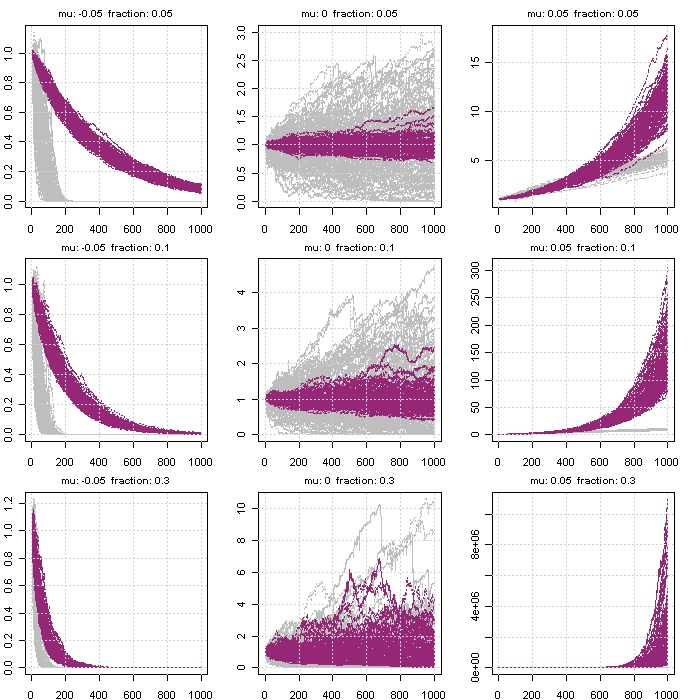## The unknown world of size

You think timing is everything in markets? Think twice!

Timing is not everything. Size matters!
Typically investors size their trades arbitrarily and notional stays fixed until exit. When losses occur they increase the size of the position to reduce the average cost of the trade. In effect they employ a small martingale game.

In martingale games, the size is increased at each subsequent loss until gains are realized. However, the strategy generates enormous bets and eventually disaster.

The opposite is an anti-martingale strategy where the size is a proportion of portfolio equity. When this proportion is fixed (a special case) we deploy a fixed fractional strategy.

Fixed-fractional is a superior strategy. It maximizes growth on favorable games while it protects the capital otherwise.100 simulations of 1000 paths for fixed fractional (purple) and martingate (gray). Simulations of returns assume normal distribution with 10% standard deviation

Contrary to conventional thought, increasing the position size does not necessarily increase average return. There is an optimal point where average return is maximized. One should aim to reside on the left side of the bell shaped curve.

#### Assume a winning strategy where:

• Each trade has a probability to win vs. lose 51% / 49%
• Each trade there are two possible outcomes \$1 and -\$1 (bernulli distribution)
• Optimum sizing is f= (51-49)/100 = 2%
• The optimum size in order to maximize growth over the long term is 2% of portfolio equity each time

#### Assume a winning strategy where:

• Each trade follows a normal probability distribution with mean (μ) and standard deviation (σ)
• Optimum sizing1 is f = μ/σ^2
• The optimum size in order to maximize growth over the long term is f% of portfolio equity each time